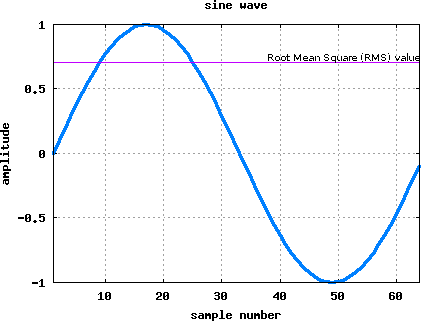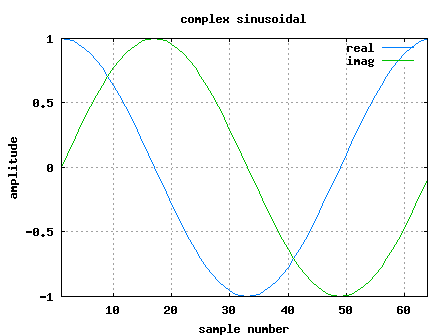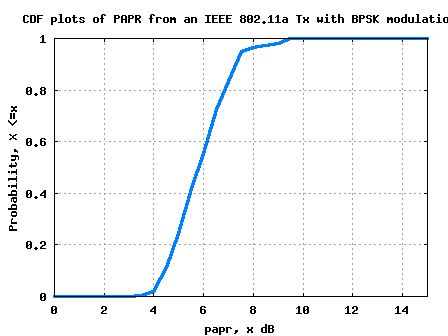- DSP log - http://www.dsplog.com -

Peak to Average Power Ratio for OFDM

Posted By Krishna Sankar On February 24, 2008 @ 7:51 am In OFDM | 272 Comments

Let us try to understand peak to average power ratio (PAPR) and its typical value in an OFDM system specified per IEEE 802.11a specifications .

## What is PAPR?

The peak to average power ratio for a signal is defined as
, where
corresponds to the conjugate operator.

Expressing in deciBels,
.

## PAPR of a single sine tone

Consider a sinusoidal signal
having the period .

The peak value of the signal is
.

The mean square value of the signal is,
.

Given so, the PAPR of a single sine tone is,
.Figure: Wave form of single sine tone

% Example Matlab/Octave script
clear all
close all
xt = sin(2*pi*1*[0:1/64:0.999]);
plot(xt,'b.-','LineWidth',4)
grid on
xlabel('sample number')
ylabel('amplitude')
title('sine wave')
meanSquareValue = xt*xt'/length(xt)
peakValue = max(xt.*conj(xt))

## PAPR of a complex sinusoidal

Consider a sinusoidal signal
having the period .

The peak value of the signal is
.

The mean square value of the signal is,
.

Given so, the PAPR of a single complex sinusoidal tone is,
.Figure: Waveform a single complex sinusoidal

close all
clear all
% defining a signal in frequency domain
% subcarrier +1 alone
xF = [zeros(1,6) zeros(1,26) 0 1 zeros(1,25) zeros(1,5) ];
xt = 64*ifft(fftshift(xF));
meanSquareValue = xt*xt'/length(xt)
peakValue = max(xt.*conj(xt))
plot(real(xt),'b','LineWidth',2)
hold on
plot(imag(xt),'g','LineWidth',2)
xlabel('sample number')
ylabel('amplitude')
title('complex sinusoidal')
legend('real', 'imag')
grid on

## Maximum expected PAPR from an OFDM waveform

From the previous post (here), we have learned that an OFDM signal is the sum of multiple sinusoidals having frequency seperation where each sinusoidal gets modulated by independent information . Mathematically, the transmit signal is,

For simplicity, let us assume that for all the subcarriers. In that scenario, the peak value of the signal is,
.

The mean square value of the signal is,
.

Given so, the peak to average power ratio for an OFDM system with subcarriers and all subcarriers are given the same modulation is,
.

It is reasonably intuitive that the above value corresponds to the maximum value of PAPR (when all the subcarriers are equally modulated, all the subcarriers align in phase and the peak value hits the maximum).

## PAPR in IEEE 802.11a OFDM transmission

Per the IEEE 802.11a specifications , we have used subcarriers. Given so, the maximum expected PAPR is 52 (around 17dB!!). However, thanks to the scrambler, all the subcarriers in an OFDM symbol being equally modulated is unlikely.

Using a small script, the cumulative distribution of PAPR from each OFDM symbol, modulated by a random BPSK signal is obtained.Figure: Cumulative distribution (CDF) plot of PAPR from a random BPSK signal

As can be observed, the observed PAPR seems to be distributed from around +3.5dB to a maximum value of 10dB.

Hope this helps.
Krishna